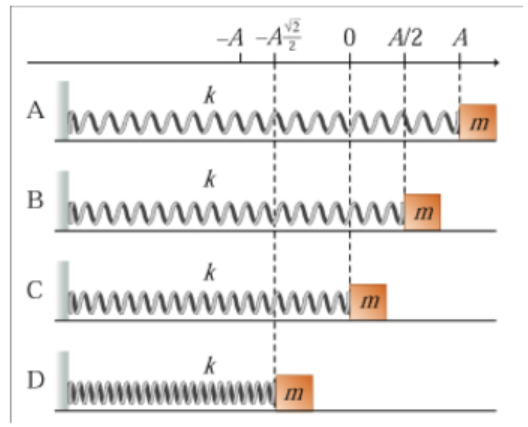# Problem:  Learning Goal: To learn to apply the law of conservation of energy to the analysis of harmonic oscillators.Systems in simple harmonic motion, or harmonic oscillators, obey the law of conservation of energy just like all other systems do. Using energy considerations, one can analyze many aspects of motion of the oscillator.Find the kinetic energy K of the block at the moment labeled B. Express your answer in terms of k and A.

###### FREE Expert Solution

The mechanical energy of an SHM oscillator:

$\overline{)\frac{\mathbf{1}}{\mathbf{2}}{\mathbf{k}}{{\mathbf{A}}}^{{\mathbf{2}}}{\mathbf{=}}\frac{\mathbf{1}}{\mathbf{2}}{\mathbf{k}}{{\mathbf{x}}}^{{\mathbf{2}}}{\mathbf{+}}\frac{\mathbf{1}}{\mathbf{2}}{\mathbf{m}}{{{\mathbf{v}}}_{{\mathbf{x}}}}^{{\mathbf{2}}}}$where (1/2)kA2 is the total energy E, (1/2)kx2 is the potential energy U at a point x, and (1/2)mvx2 is the kinetic energy K.

89% (32 ratings)###### Problem Details

Learning Goal: To learn to apply the law of conservation of energy to the analysis of harmonic oscillators.

Systems in simple harmonic motion, or harmonic oscillators, obey the law of conservation of energy just like all other systems do. Using energy considerations, one can analyze many aspects of motion of the oscillator.Find the kinetic energy K of the block at the moment labeled B. Express your answer in terms of k and A.